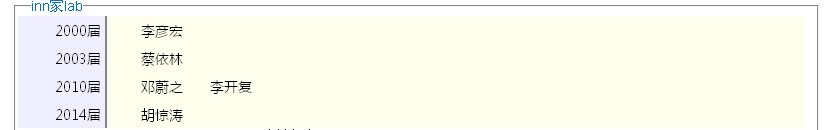php排序算法实例分析```<?php
\$array = \$mysql->query_array(\$mysql->sql_select("user","userid,truename,year"," ")); //从数据库里面读出数据
\$year = array(); //设置空数组
foreach(\$array as \$value)
{
array_push(\$year,\$value); //把数据数组里面的时间数据入栈
}
\$year = array_unique(\$year); //去除数组中重复的数据
usort(\$year, "strnatcmp"); //将数组中的数据按自然数排序
\$num = count(\$year); //统计数据中数据的个数
/*处理函数*/
function isyear(\$value,\$array)
{
\$user = array(); //建立空数组
foreach(\$array as \$val)
{
if(\$val == \$value)
{
array_push(\$user,\$val);
}
}
return \$user; //返回数组
}
?>

```

```<?php
for(\$i=0;\$i<\$num;\$i++)
{
echo "<div class='box_user'>";
echo "<div class='boxleft'>{\$year[\$i]}届</div>";
echo  "<div class='boxright'>";
\$user = isyear(\$year[\$i],\$array);
foreach(\$user as \$v)
{
echo "<span class={\$v}>{\$v}</span>";
}
echo "</div>";
echo "</div>";
}
?>

```

```<?php
memory_get_usage();
?>

```

php性能分析之php-fpm慢执行日志slow log用法浅析

php实现跨域提交form表单的方法【2种方法】